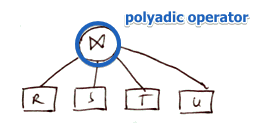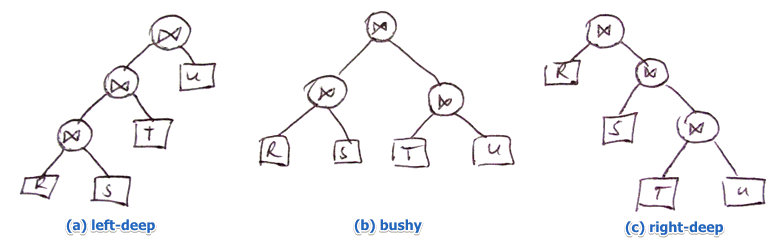# ML Wiki

## Join Ordering

This is a part of Physical Query Plan Optimization procedure

In a Logical Query Plan the order of joins is not fixed

• there we assumed that this is a polyadic operation

Example

• $R(A, B), S(B, C), T(C, D), U(D, A)$
• want $R \Join S \Join T \Join U$
•## Importance of Orderings

But physical join operators are binary!

• Order becomes important

how can we order these joins?

• $R \Join S \Join T \Join U \equiv$
• $((R \Join S) \Join T) \Join U \equiv$
• $(R \Join S) \Join (T \Join U) \equiv$
• $((R \Join T) \Join U) \Join S \equiv$
• $...$
• A lot! (note that natural join is possible even if there are no matching tuples)

Recall that Join is the most expensive operation

• need to carefully choose the order

### Example

• given: $R(A, B), S(B, C), T(A, E)$
• statistics:
• $B(R) = 50, B(S) = 50, B(T) = 50$
• $B(R \Join S) = 150$
• $B(S \Join T) = 2500$ ($S$ and $T$ don't have anything in common - so it's a cartesian product)
• $B(R \Join T) = 200$
• assume ideal case: everything can be done with one-pass join algorithm
• i.e. we have # of free buffers $M = 51$
• what's the best ordering for $R \Join S \Join T$?

$R \Join (S \Join T)$

• $S \Join T$ - the largest
• cost:
• $B(R) +$ (read $B$ once)
• $B(S \Join T) +$ (too big intermediate result - need to flush to disk)
• $B(S) + B(T)$
• = 2650

$S \Join (R \Join T)$

• cost: $B(S) + B(R \Join T) + B(R) + B(T) = 350$

$(R \Join S) \Join T$

• cost: $B(T) + B(R \Join S) + B(R) + B(S) = 300$

So we see that the order is indeed important

• we want to avoid computing big subresults that we don't need afterwards

## Optimization Problem

### Possibly Orderings

We see that we need to enumerate all possible join orderings

• in how many ways we can put ()s?
• how many permutations are there?

$\Rightarrow$ the number of possible orderings is $n! \times T(n)$

• $n!$ - number of ways to permute $n$ relations
• $T(n)$ ways to create a binary tree over $n$ leaf nodes
• $T(1) = 1, T(n) = \sum_{i = 1}^{n - 1} T(i) \times T(n - 1)$

The resulting space is super-exponential:

 $n$ $n! \times T(n)$ 2 3 4 5 6 7 8 2 12 120 1680 30 240 665 580 17 297 280

The query optimization must not take longer than the most stupid and naive way of executing it

• so we disregard the option of trying all possible orderings and infeasible

### Types of Ordering

Instead of listing all possible orderings we will consider only one of the following types• (a): $((R \Join S) \Join T) \Join U$
• (b): $(R \Join S) \Join (T \Join U)$
• (c): $R \Join (S \Join (T \Join U))$

a typical query optimizer usually looks only at left-deep join orderings

• (a) $\approx$ (c), but there are subtle implementation-wise differences
• (like being able to pipeline some results, etc)
• still, there are $n!$ possible orderings (and it's still exponential)

This is an Optimization Problem. Solutions:

## Greedy Algorithm

Always make locally optimal choices

Algorithm

• for remaining relations choose the cheapest relation to join with the result-so-far
• repeat until there are no relations left

That generates a left-deep join ordering

### Not Always Optimal

Of course since it uses some kind of Local Search, it may stuck in local optima

Suppose:

• join on $R(A, B), S(B, C), T(C, D), U(A, D)$
• costs:
• $\underbrace{B(R \Join S) = 100}_{(1)}, \underbrace{B((R \Join S) \Join T)) = 2000}_{(2)}$
• $\underbrace{B(R \Join U) = 200}_{(3)}, \underbrace{B((R \Join U) \Join T)) = 1000}_{(4)}$
• Greedy algorithm will select
• $((R \Join S) \Join T) \Join U$
• $(1)$ gives us better cost than $(3)$
• alternative ordering:
• $((R \Join U) \Join T) \Join S$
• $(3)$ is not better than $(1)$, but $(4)$ (given $(3)$) is better than $(2)$ (given $(1)$)
• 900 I/Os saved!

## Exercises

Main Article: Query Plan Selection Exercises